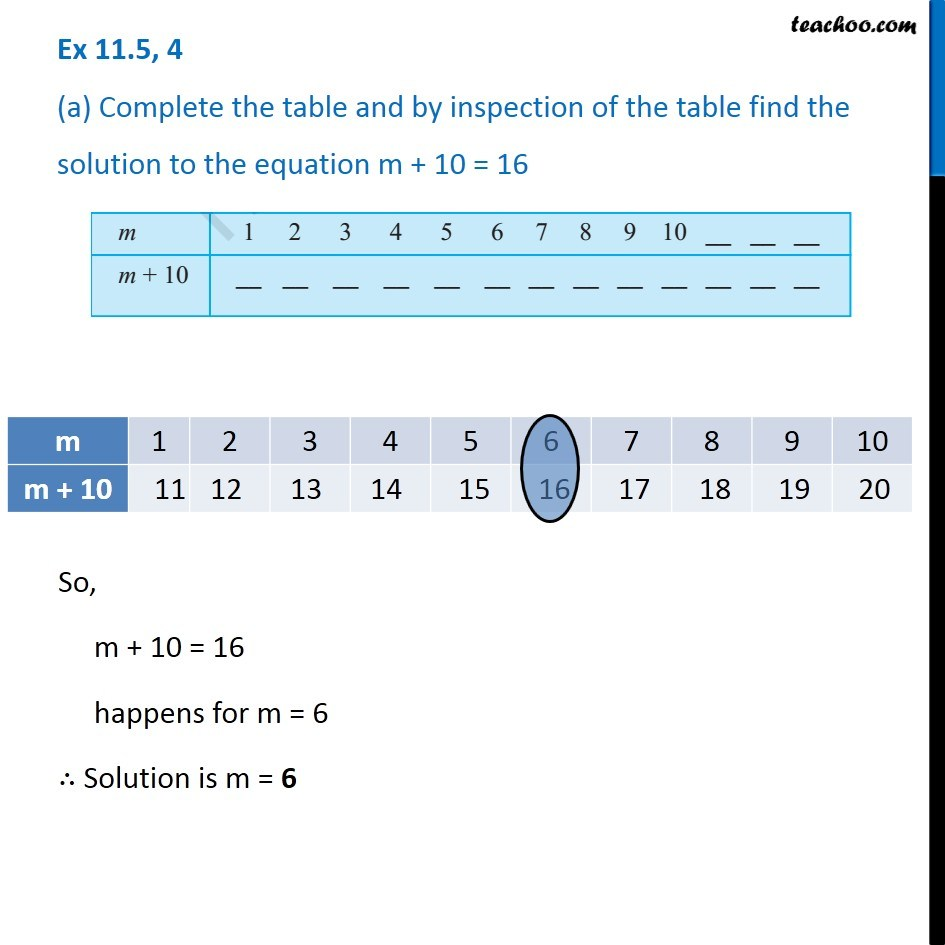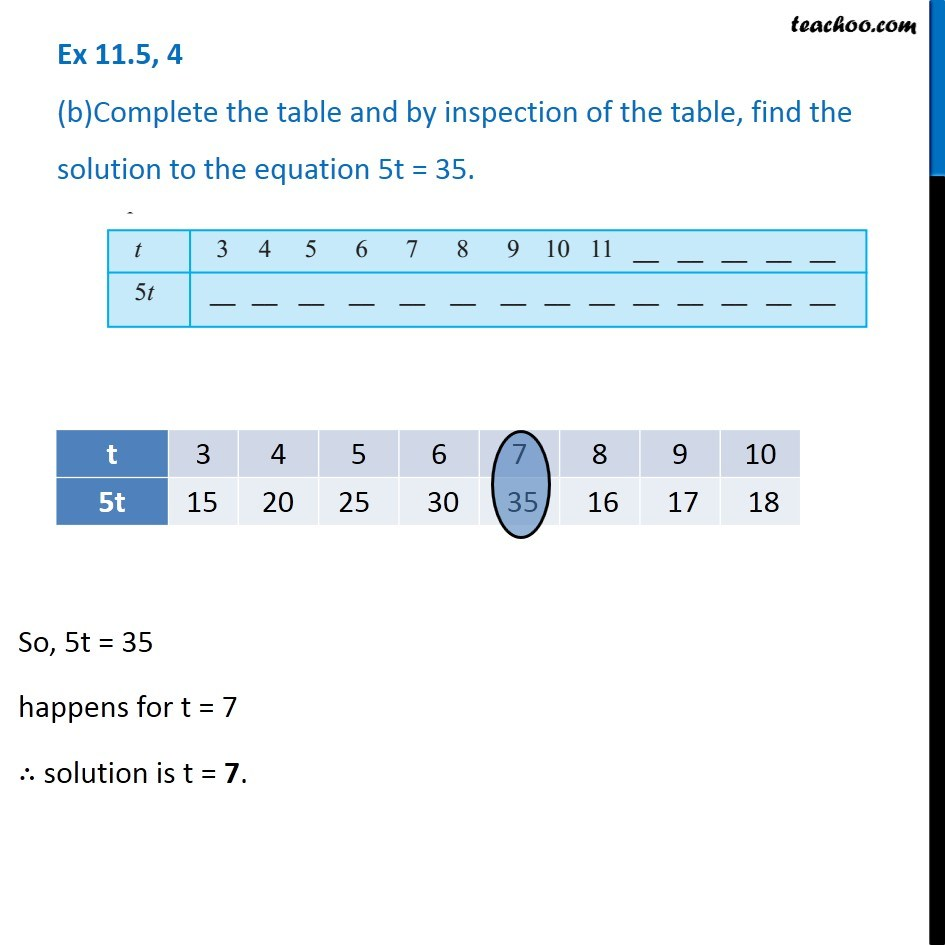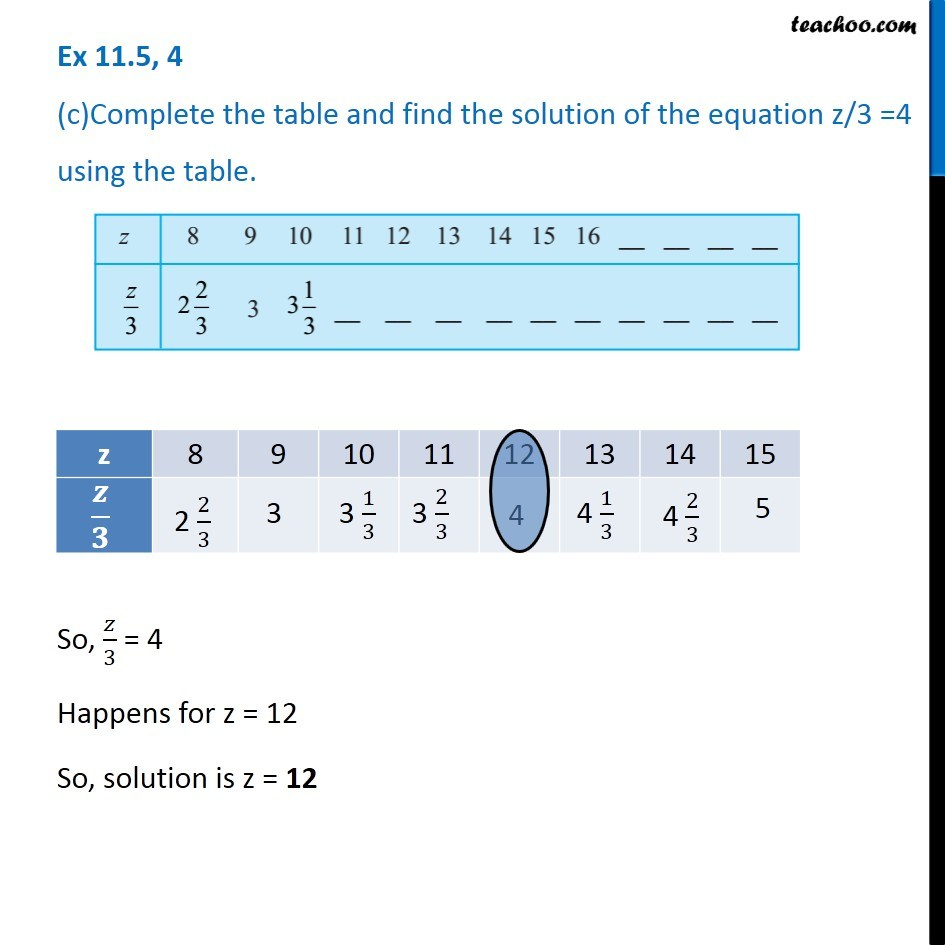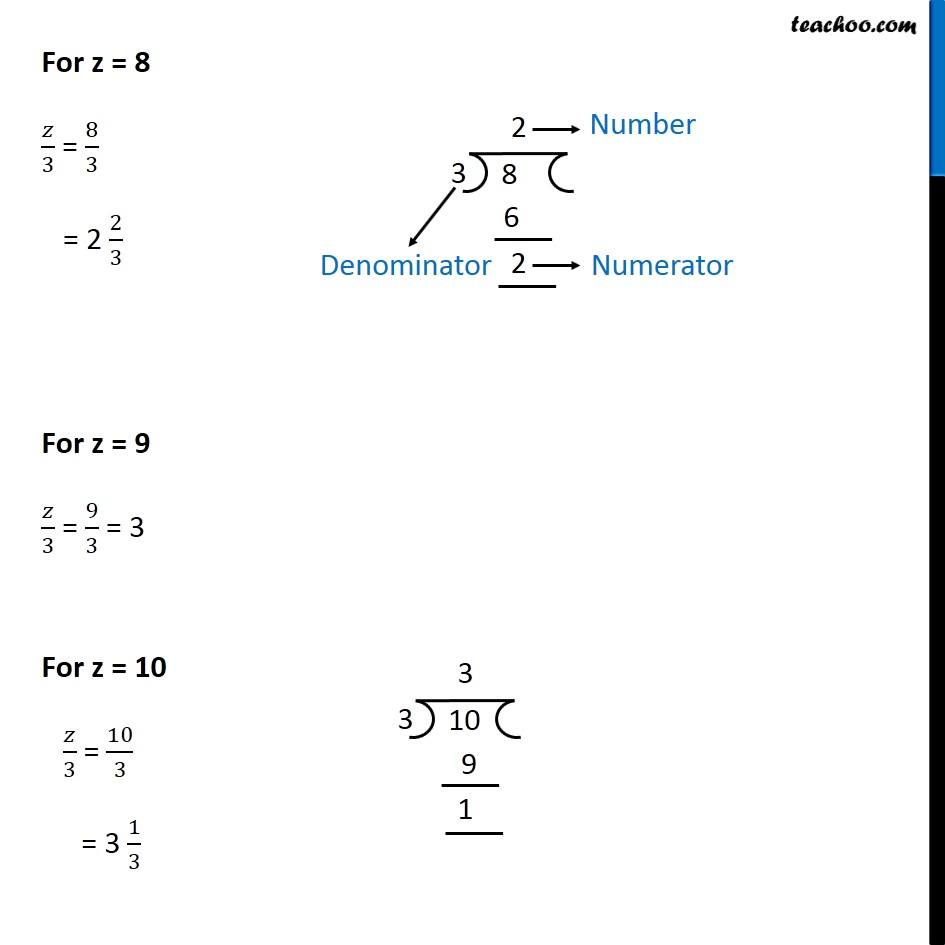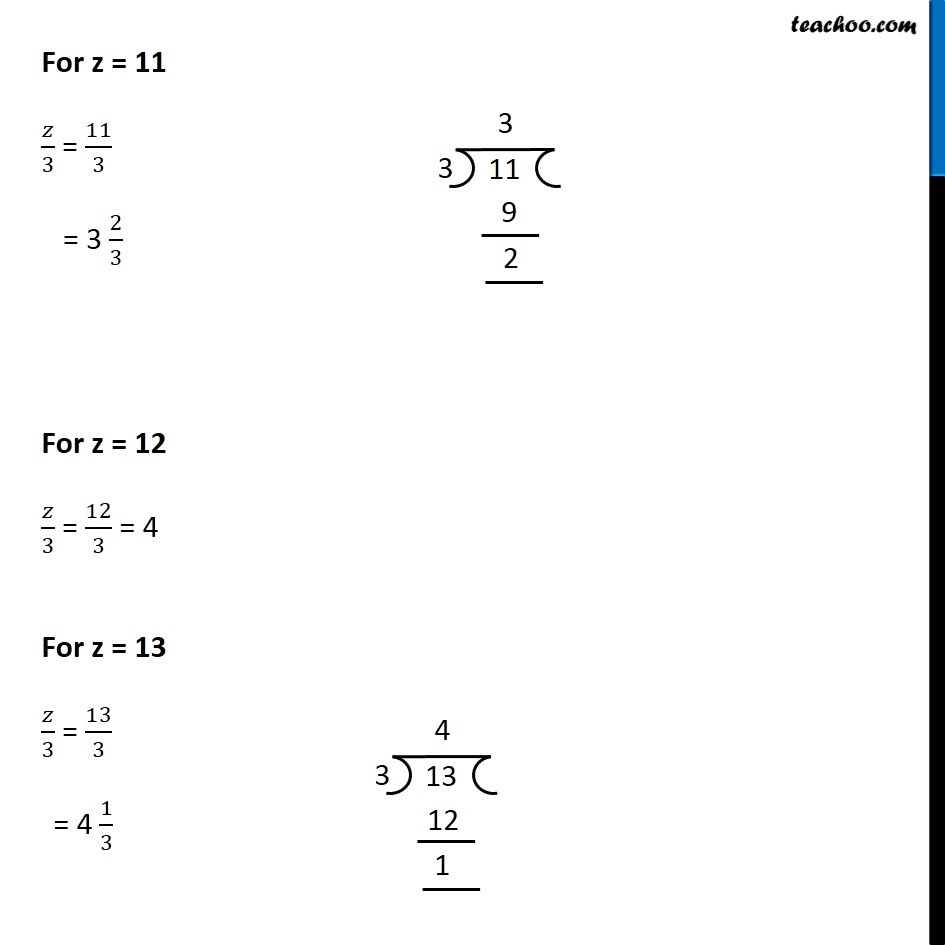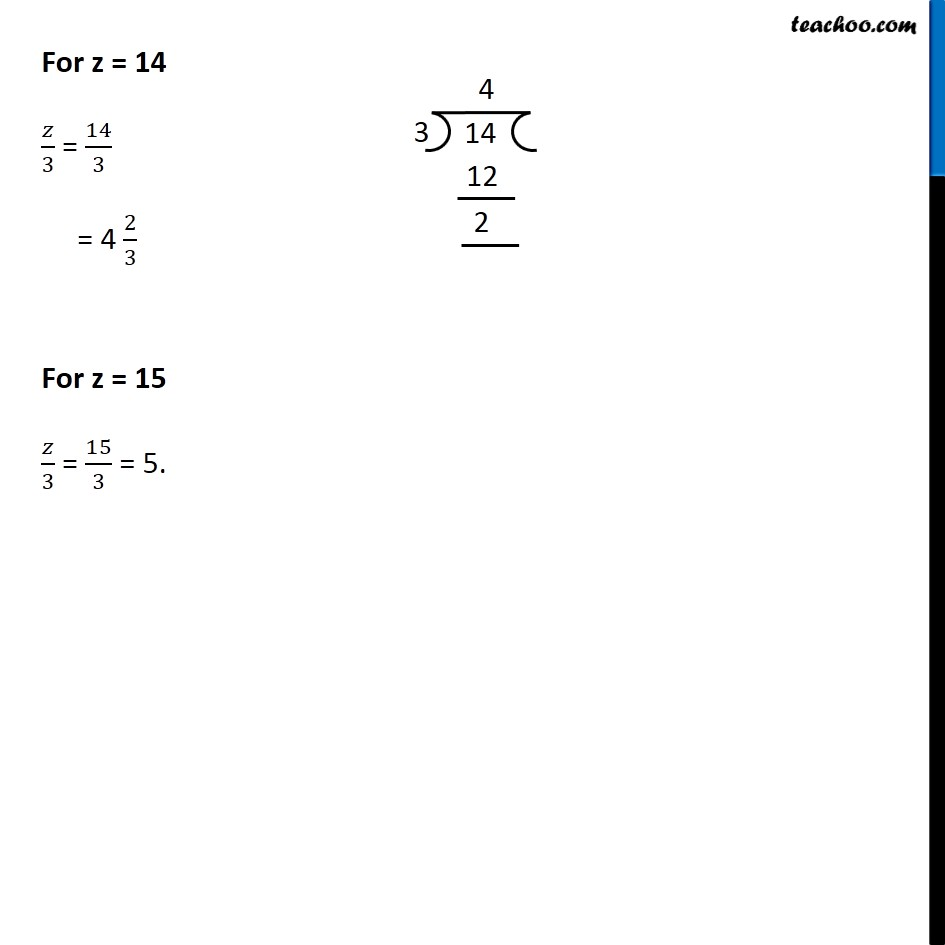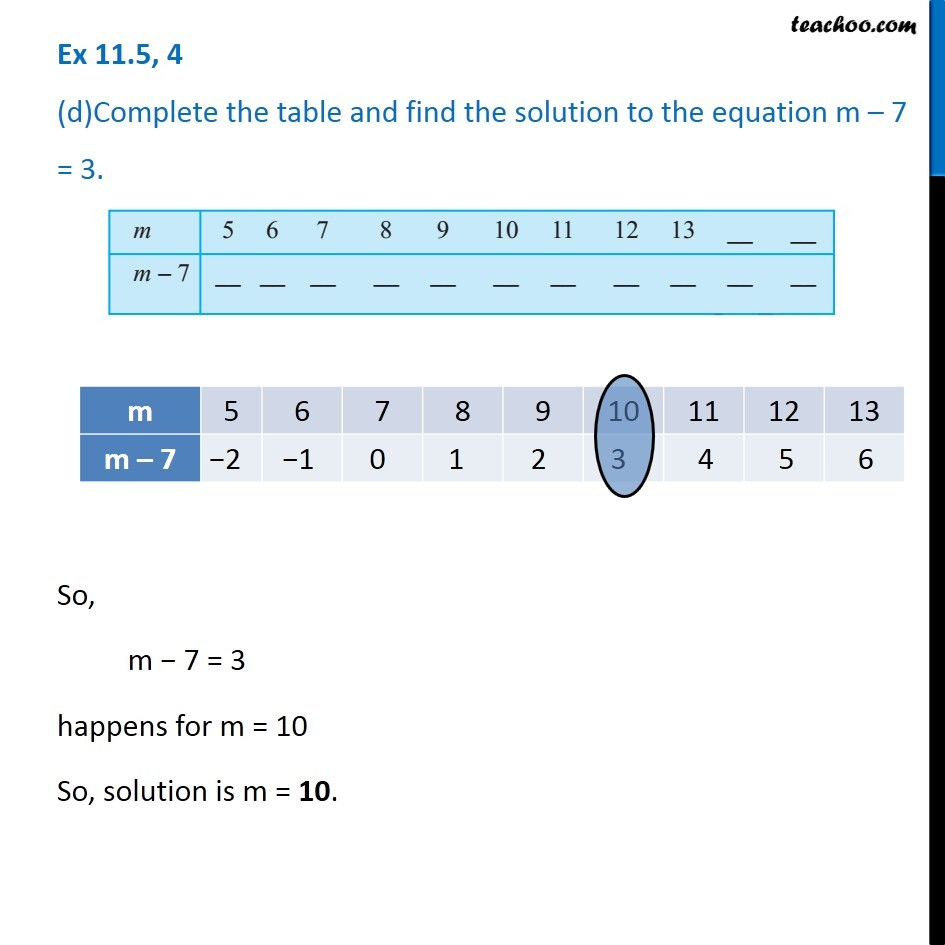Subscribe to our Youtube Channel - https://you.tube/teachoo

1. Chapter 11 Class 6 Algebra
2. Concept wise
3. Solution of equations

Transcript

Ex 11.5, 4 (a) Complete the table and by inspection of the table find the solution to the equation m + 10 = 16So, m + 10 = 16 happens for m = 6 ∴ Solution is m = 6 Ex 11.5, 4 (b)Complete the table and by inspection of the table, find the solution to the equation 5t = 35. So, 5t = 35 happens for t = 7 ∴ solution is t = 7. Ex 11.5, 4 (c)Complete the table and find the solution of the equation z/3 =4 using the table.So, 𝑧/3 = 4 Happens for z = 12 So, solution is z = 12 For z = 8 𝑧/3 = 8/3 = 2 2/3 For z = 9 𝑧/3 = 9/3 = 3 For z = 10 𝑧/3 = 10/3 = 3 1/3For z = 11 𝑧/3 = 11/3 = 3 2/3 For z = 12 𝑧/3 = 12/3 = 4 For z = 13 𝑧/3 = 13/3 = 4 1/3For z = 14 𝑧/3 = 14/3 = 4 2/3 For z = 15 𝑧/3 = 15/3 = 5. Ex 11.5, 4 (d)Complete the table and find the solution to the equation m – 7 = 3.So, m − 7 = 3 happens for m = 10 So, solution is m = 10.

Solution of equations